Convenient and smart calculator with built-in function that can use various calculator in
one app and prevent user’s mistake as much as possible

★ Convenient and smart calculator with built-in function that can use various calculator in
one app and prevent user’s mistake as much as possible
★ History, memory storage function, Undo, Redo function
★ Supports 7 different calculator themes
★ Calculator button sound and font change function

• Integrated calculator configuration –

1) Simple Calculator
1) General Calculator
2) Engineering Calculator
3) Statistical calculator
4) Precision Calculator
5) Date Calculator
6) Unit conversion
7) Percent CalculatorInterest calculator (deposit / savings / loan)

WHAT’S NEW

☞ ver 9.2
→ Modify date calculator calendar selection function
→ Fixed errors related to interest calculator keyboard

Screenshots

•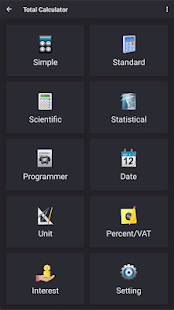•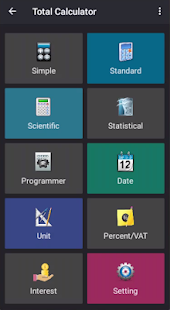•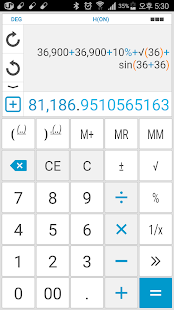•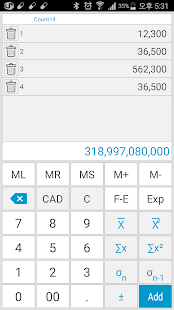•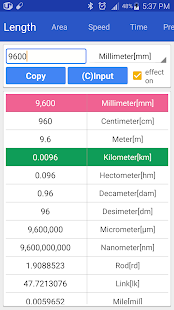•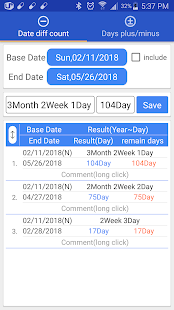•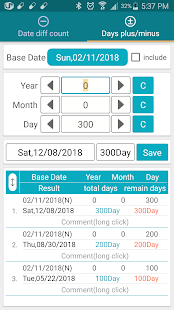••